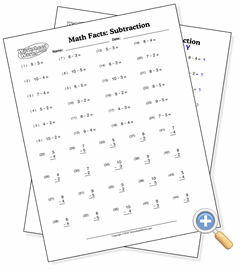# Subtraction from TenDevelop subtraction skills starting from numbers up to ten

This worksheet presents subtraction problems that start with values as high as ten. The answer to each problem will be determined by which numbers you want to subtract with so that the result is never below zero.